# Boolean Expressions to Make Comparisons Boolean expression Represents

• Slides: 61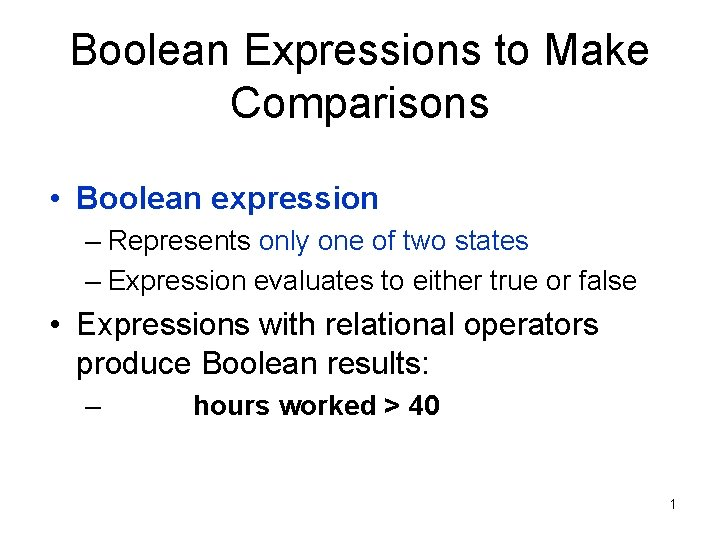Boolean Expressions to Make Comparisons • Boolean expression – Represents only one of two states – Expression evaluates to either true or false • Expressions with relational operators produce Boolean results: – hours worked > 40 1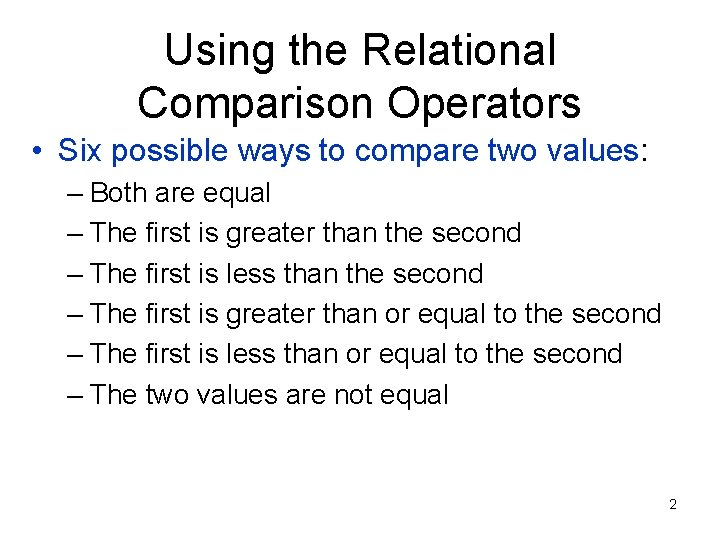Using the Relational Comparison Operators • Six possible ways to compare two values: – Both are equal – The first is greater than the second – The first is less than the second – The first is greater than or equal to the second – The first is less than or equal to the second – The two values are not equal 2Relational Comparison Operators • Relational comparison operators: – To express Boolean tests when comparing values • Different languages use different symbols – – – Equals: = Less than: < Greater than: > Less than or equal: <= Greater than or equal: >= Not equal: <> 3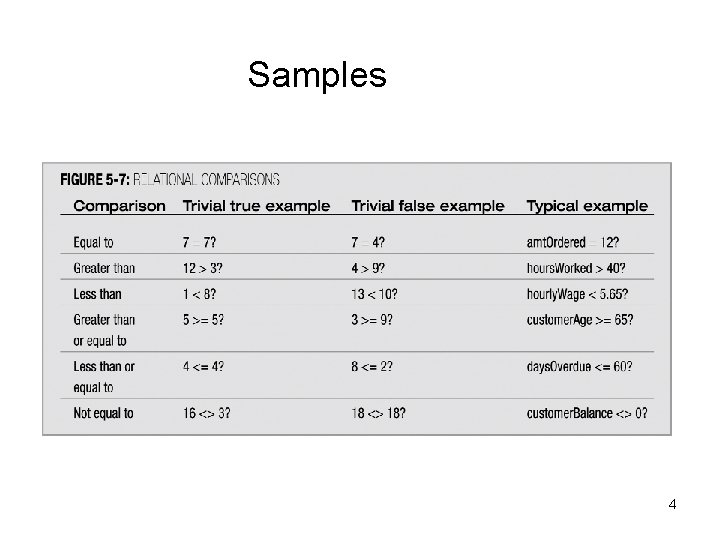Samples 4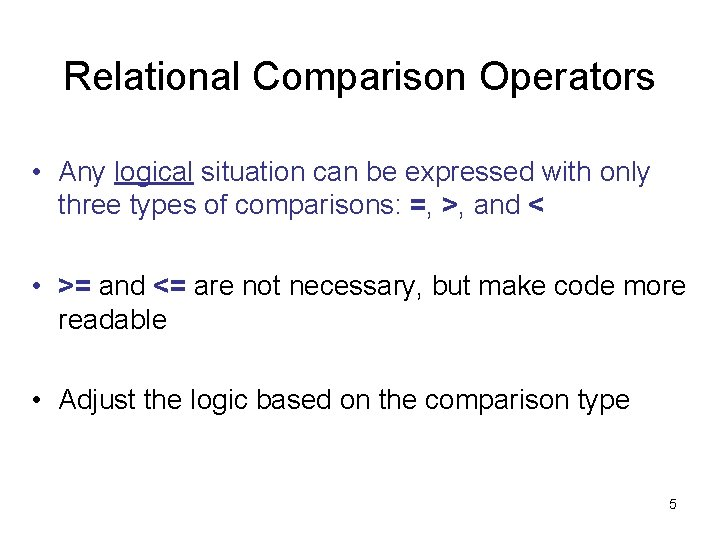Relational Comparison Operators • Any logical situation can be expressed with only three types of comparisons: =, >, and < • >= and <= are not necessary, but make code more readable • Adjust the logic based on the comparison type 5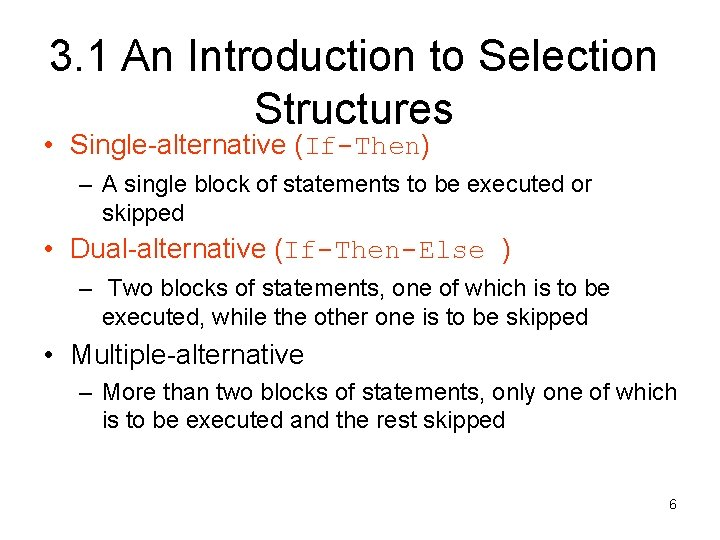3. 1 An Introduction to Selection Structures • Single-alternative (If-Then) – A single block of statements to be executed or skipped • Dual-alternative (If-Then-Else ) – Two blocks of statements, one of which is to be executed, while the other one is to be skipped • Multiple-alternative – More than two blocks of statements, only one of which is to be executed and the rest skipped 6Evaluating Boolean Expressions to Make Comparisons • Dual-alternative (or binary) selection structure: – Provides an action for each of two possible outcomes 7Evaluating Boolean Expressions to Make Comparisons (continued) • Dual-alternative (or binary) selection structure: – Also called an if-then-else structure 8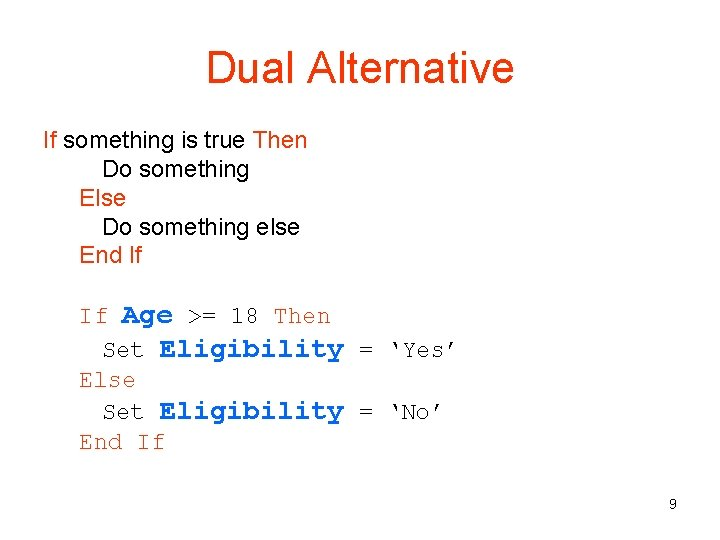Dual Alternative If something is true Then Do something Else Do something else End If If Age >= 18 Then Set Eligibility = ‘Yes’ Else Set Eligibility = ‘No’ End If 9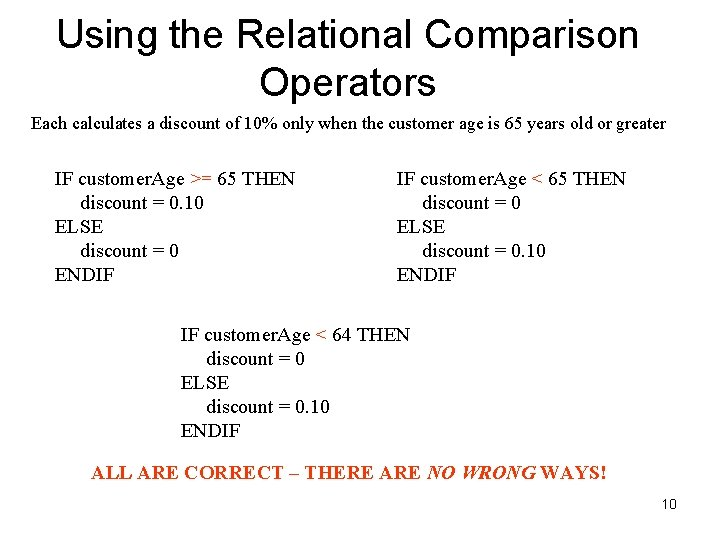Using the Relational Comparison Operators Each calculates a discount of 10% only when the customer age is 65 years old or greater IF customer. Age >= 65 THEN discount = 0. 10 ELSE discount = 0 ENDIF IF customer. Age < 65 THEN discount = 0 ELSE discount = 0. 10 ENDIF IF customer. Age < 64 THEN discount = 0 ELSE discount = 0. 10 ENDIF ALL ARE CORRECT – THERE ARE NO WRONG WAYS! 10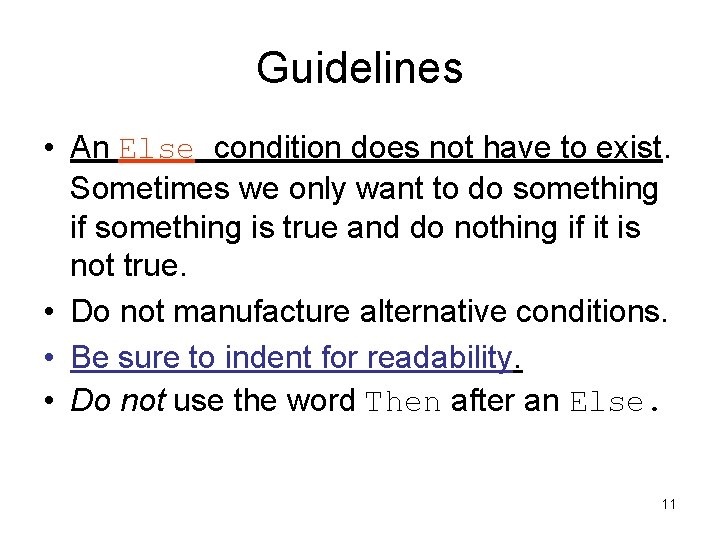Guidelines • An Else condition does not have to exist. Sometimes we only want to do something if something is true and do nothing if it is not true. • Do not manufacture alternative conditions. • Be sure to indent for readability. • Do not use the word Then after an Else. 11Single Alternative If something is true Then Do something (any number of statements) End If If Age >= 18 Then Set Eligibility = “Yes” Do other things… End If 12Evaluating Boolean Expressions to Make Comparisons • Single-alternative (or unary) selection structure – Action is provided for only one outcome 13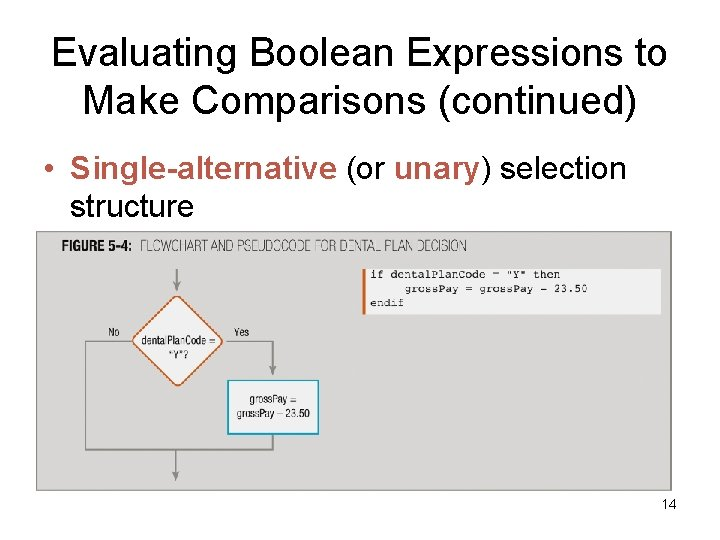Evaluating Boolean Expressions to Make Comparisons (continued) • Single-alternative (or unary) selection structure – Also called an if-then structure 14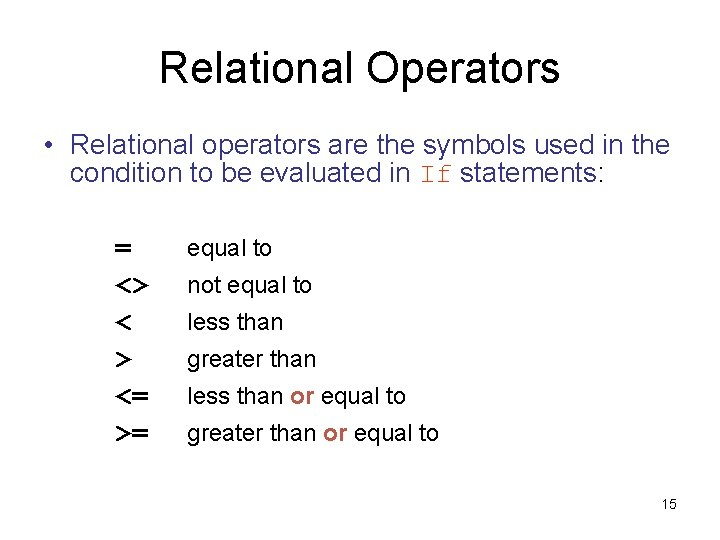Relational Operators • Relational operators are the symbols used in the condition to be evaluated in If statements: = <> < > <= >= equal to not equal to less than greater than less than or equal to greater than or equal to 15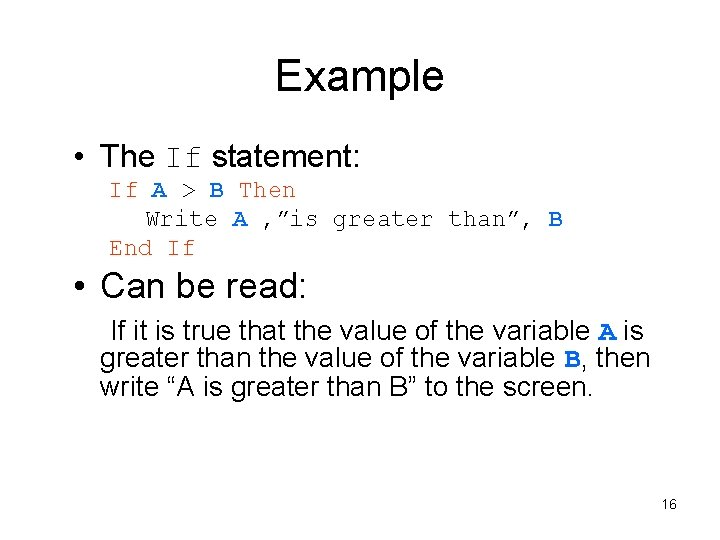Example • The If statement: If A > B Then Write A , ”is greater than”, B End If • Can be read: If it is true that the value of the variable A is greater than the value of the variable B, then write “A is greater than B” to the screen. 16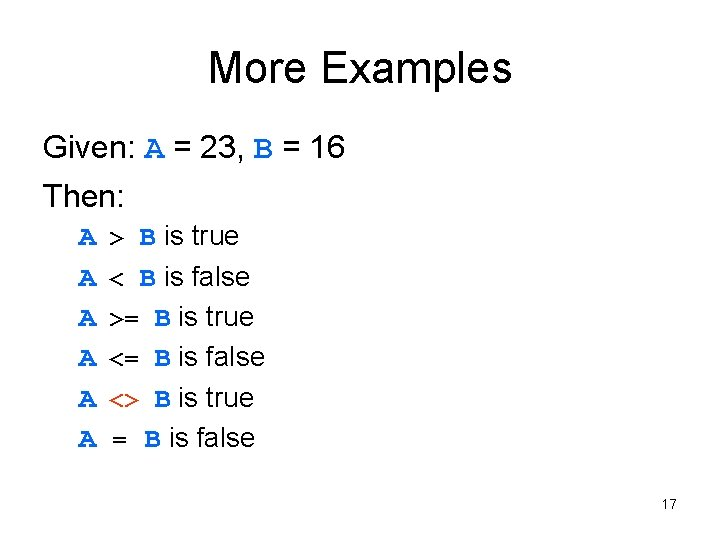More Examples Given: A = 23, B = 16 Then: A A A > B is true < B is false >= B is true <= B is false <> B is true = B is false 17Comparison vs. Assignment Operators The equals sign (=) in this text may have two different meanings. The difference is very significant. As an assignment operator, the equals sign sets the value of an expression on the right side to the variable on the left side. As a comparison operator, the equals sign asks the question, “Is the value of the variable on the left side the same as the value of the expression, number, or variable on the right side? ” Many programming languages distinguish between these two operators as follows: • a single equals sign (=) signifies the assignment operator • a double equals sign (==) signifies the comparison operator This is demonstrated in the examples that follow in the next slides. 18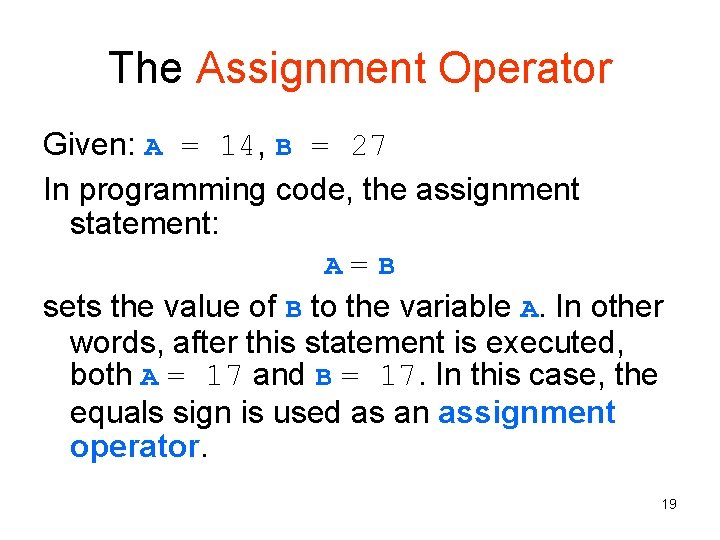The Assignment Operator Given: A = 14, B = 27 In programming code, the assignment statement: A=B sets the value of B to the variable A. In other words, after this statement is executed, both A = 17 and B = 17. In this case, the equals sign is used as an assignment operator. 19The Comparison Operator Given: A = 14, B = 27 Using the relational operators, the statement: A == B is a comparison. This statement asks the question, “Is the value of A the same as the value of B? ” In this case, since A and B have different values, the answer is “no” and the statement would result in a value of False. In this text, we often use the one symbol (=) to represent both assignment and comparison operators and rely on the context to make the meaning clear. 20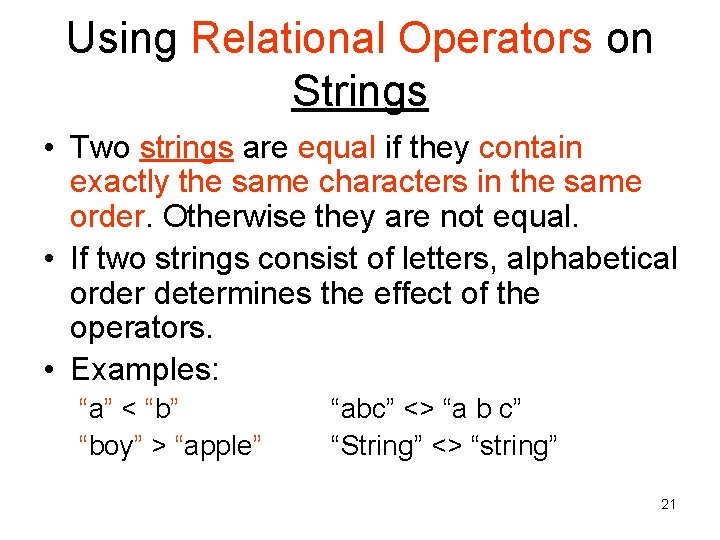Using Relational Operators on Strings • Two strings are equal if they contain exactly the same characters in the same order. Otherwise they are not equal. • If two strings consist of letters, alphabetical order determines the effect of the operators. • Examples: “a” < “b” “boy” > “apple” “abc” <> “a b c” “String” <> “string” 21Logical Operators • Logical operators are used to connect simple conditions into a more complex condition called a compound condition. • The simple conditions each contain one relational operator. • Using compound conditions reduces the amount of code that must be written. 22Combining Decisions in an AND Selection • Logical AND operator: – Allows you to ask two or more questions (Boolean expressions) in a single comparison – Each Boolean expression in an AND selection must be true to produce a result of true – Question placed first will be asked first, so consider efficiency 23Understanding AND Logic • AND decision – Requires that both (ALL) of two tests evaluate to True – Requires a nested decision (nested if) 24The AND Operator • A compound condition consisting of two simple conditions joined by an AND is true only if both simple conditions are true. It is false if even one of the conditions is false. The statement: If (X > 5) AND (X < 10) Then … is true only if X is 6, 7, 8, or 9. It has to be both greater than 5 and less than 10 at the same time. 25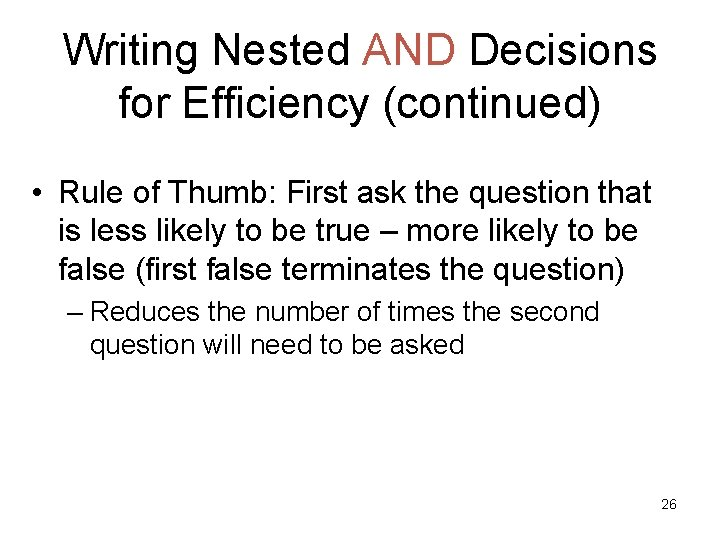Writing Nested AND Decisions for Efficiency (continued) • Rule of Thumb: First ask the question that is less likely to be true – more likely to be false (first false terminates the question) – Reduces the number of times the second question will need to be asked 26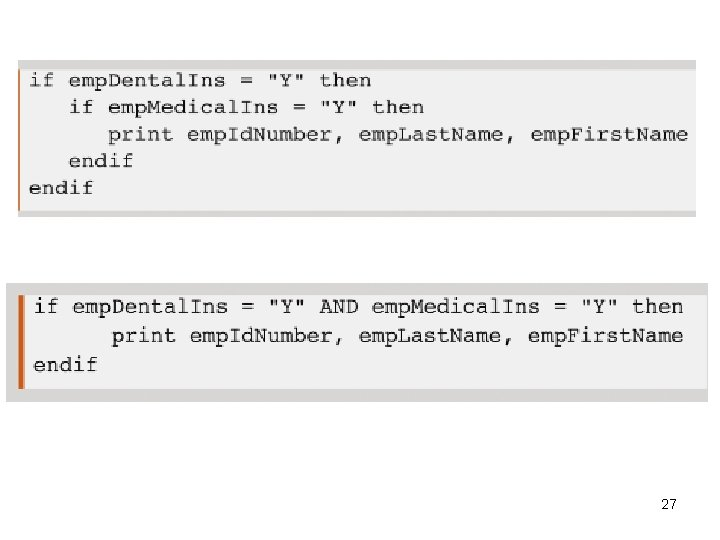27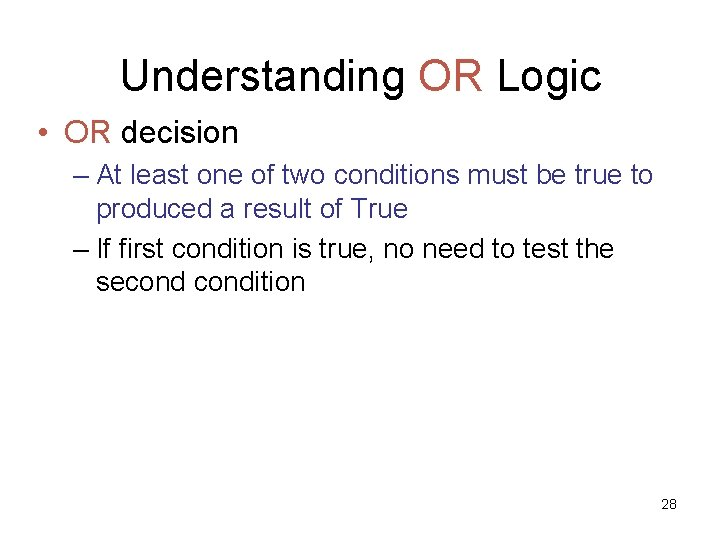Understanding OR Logic • OR decision – At least one of two conditions must be true to produced a result of True – If first condition is true, no need to test the secondition 28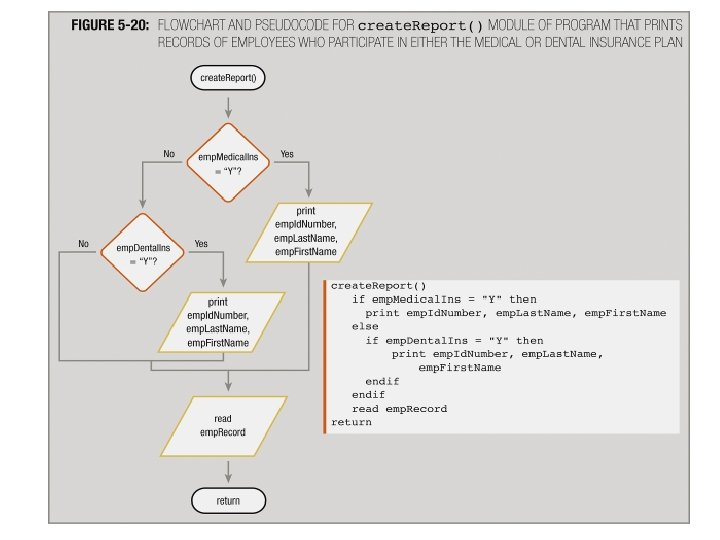29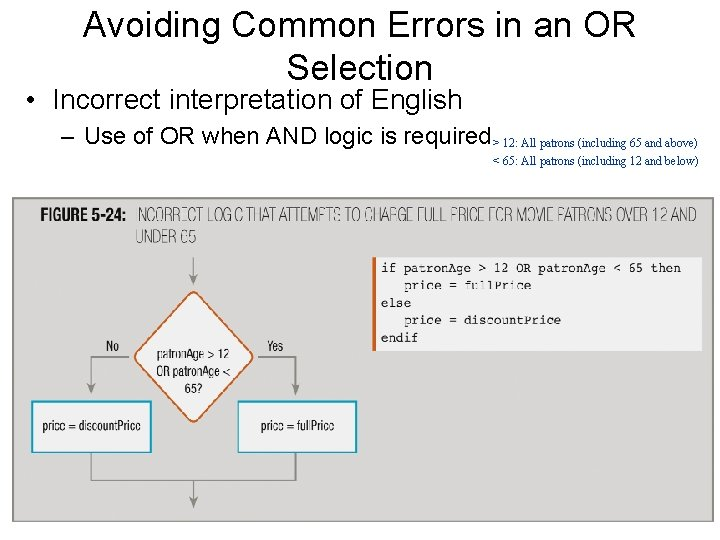Avoiding Common Errors in an OR Selection • Incorrect interpretation of English – Use of OR when AND logic is required > 12: All patrons (including 65 and above) < 65: All patrons (including 12 and below) 30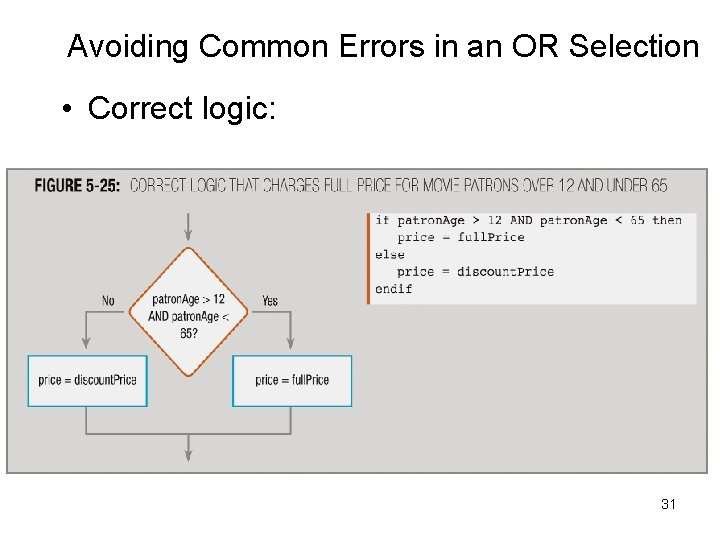Avoiding Common Errors in an OR Selection • Correct logic: 31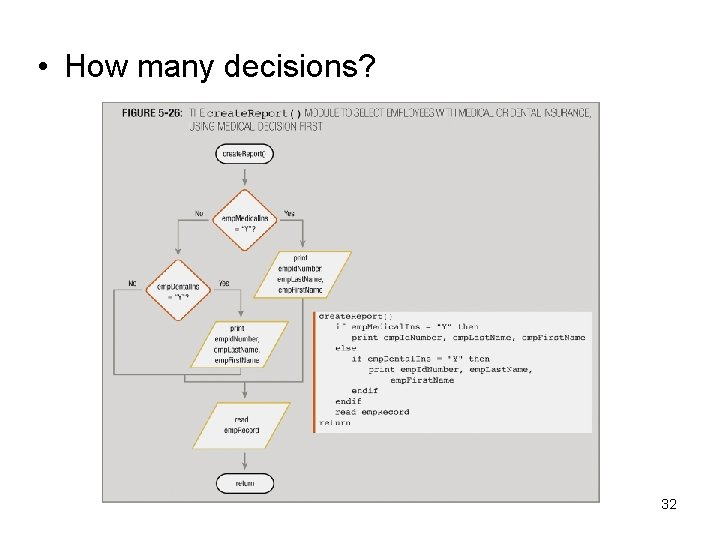• How many decisions? 32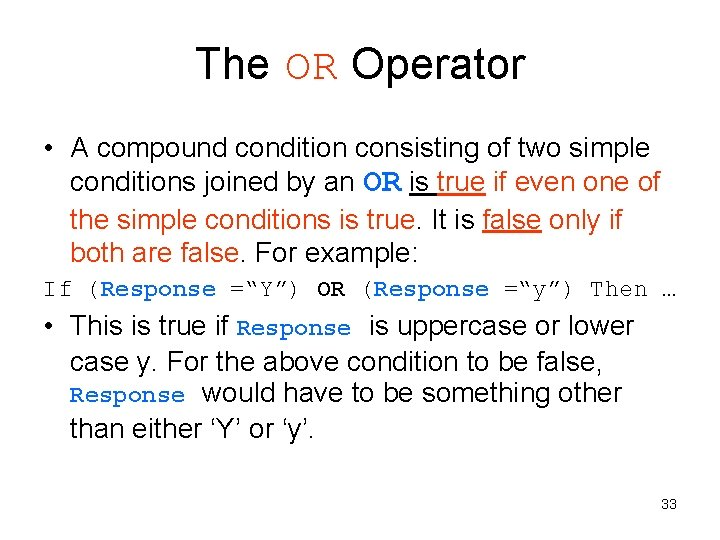The OR Operator • A compound condition consisting of two simple conditions joined by an OR is true if even one of the simple conditions is true. It is false only if both are false. For example: If (Response =“Y”) OR (Response =“y”) Then … • This is true if Response is uppercase or lower case y. For the above condition to be false, Response would have to be something other than either ‘Y’ or ‘y’. 33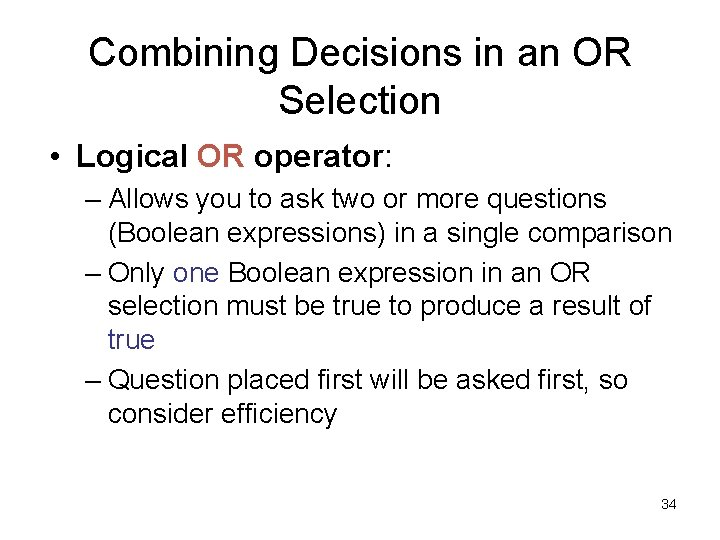Combining Decisions in an OR Selection • Logical OR operator: – Allows you to ask two or more questions (Boolean expressions) in a single comparison – Only one Boolean expression in an OR selection must be true to produce a result of true – Question placed first will be asked first, so consider efficiency 34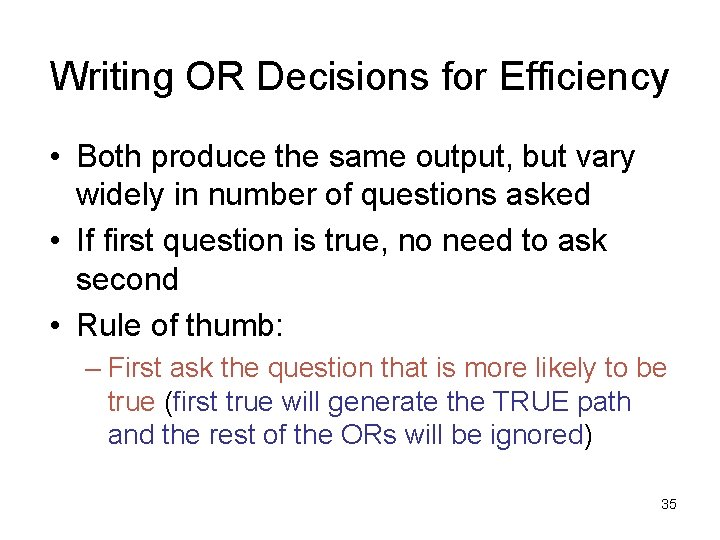Writing OR Decisions for Efficiency • Both produce the same output, but vary widely in number of questions asked • If first question is true, no need to ask second • Rule of thumb: – First ask the question that is more likely to be true (first true will generate the TRUE path and the rest of the ORs will be ignored) 35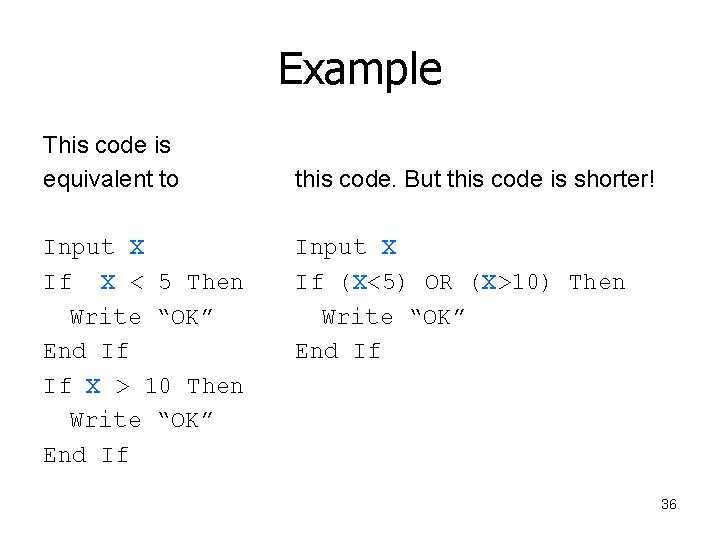Example This code is equivalent to Input X If X < 5 Then Write “OK” End If If X > 10 Then Write “OK” End If this code. But this code is shorter! Input X If (X<5) OR (X>10) Then Write “OK” End If 36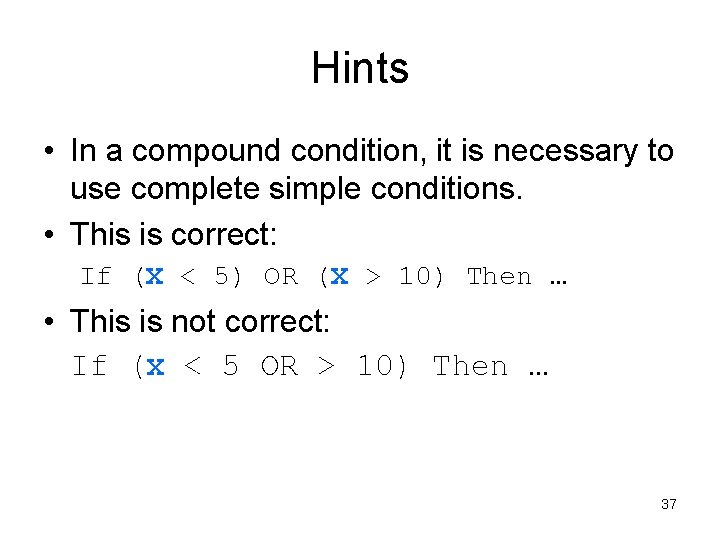Hints • In a compound condition, it is necessary to use complete simple conditions. • This is correct: If (X < 5) OR (X > 10) Then … • This is not correct: If (X < 5 OR > 10) Then … 37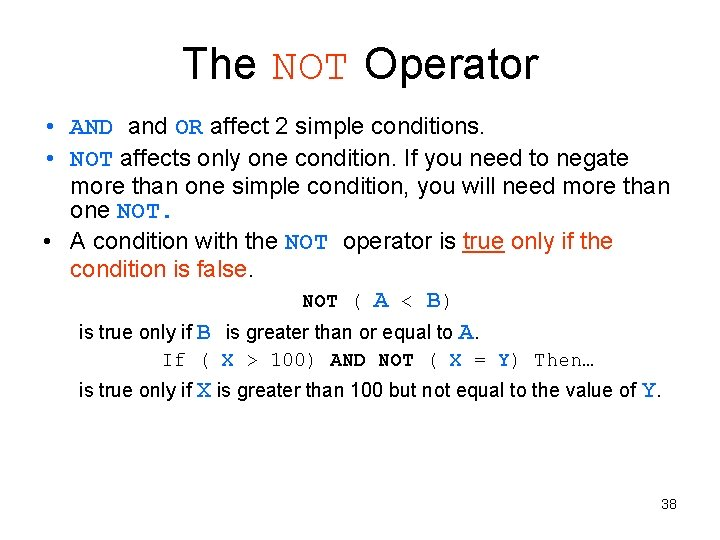The NOT Operator • AND and OR affect 2 simple conditions. • NOT affects only one condition. If you need to negate more than one simple condition, you will need more than one NOT. • A condition with the NOT operator is true only if the condition is false. NOT ( A < B) is true only if B is greater than or equal to A. If ( X > 100) AND NOT ( X = Y) Then… is true only if X is greater than 100 but not equal to the value of Y. 38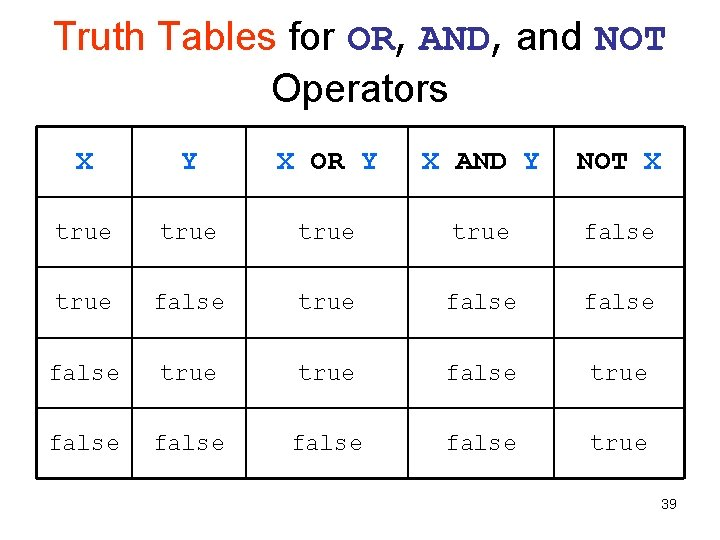Truth Tables for OR, AND, and NOT Operators X Y X OR Y X AND Y NOT X true false true false false true 39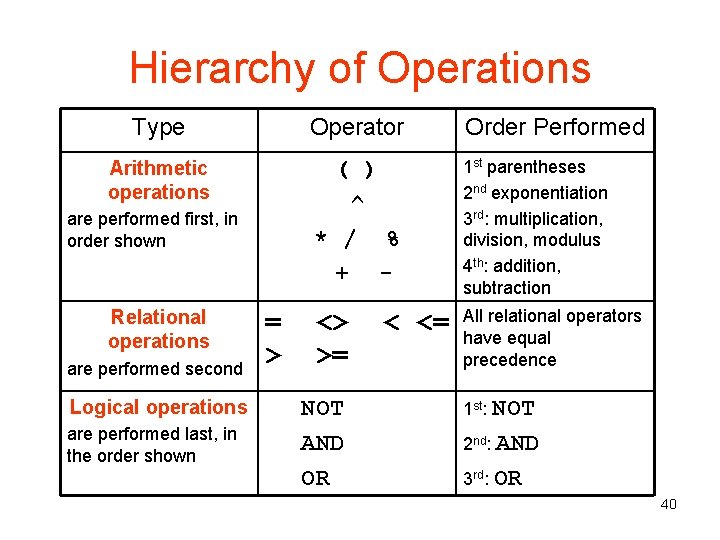Hierarchy of Operations Type Operator Order Performed Arithmetic operations ( ) ^ * / % + - 1 st parentheses 2 nd exponentiation 3 rd: multiplication, division, modulus 4 th: addition, subtraction <> >= All relational operators have equal precedence are performed first, in order shown Relational operations are performed second Logical operations are performed last, in the order shown = > NOT AND OR < <= 1 st: NOT 2 nd: AND 3 rd: OR 40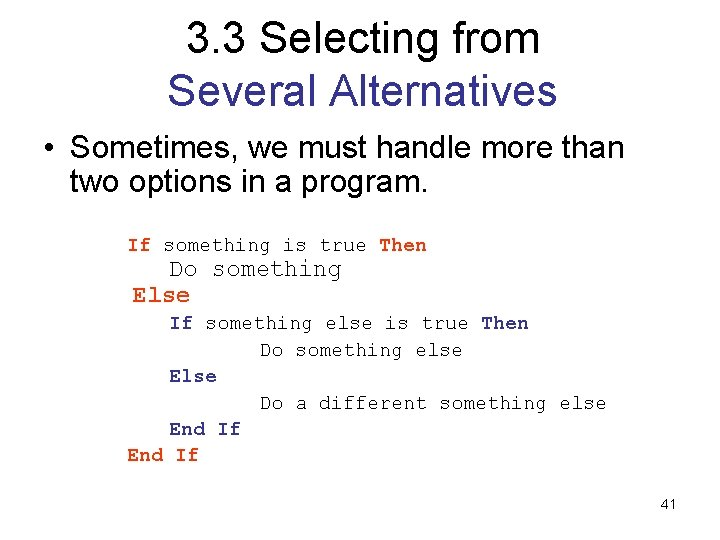3. 3 Selecting from Several Alternatives • Sometimes, we must handle more than two options in a program. If something is true Then Do something Else If something else is true Then Do something else Else Do a different something else End If 41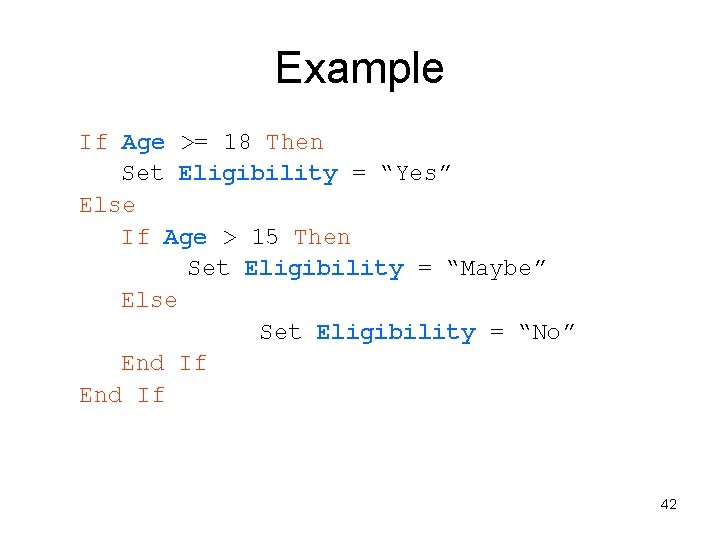Example If Age >= 18 Then Set Eligibility = “Yes” Else If Age > 15 Then Set Eligibility = “Maybe” Else Set Eligibility = “No” End If 42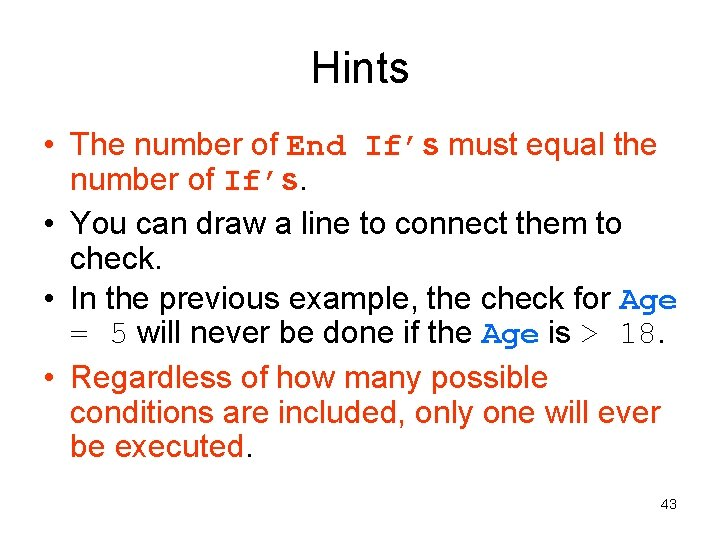Hints • The number of End If’s must equal the number of If’s. • You can draw a line to connect them to check. • In the previous example, the check for Age = 5 will never be done if the Age is > 18. • Regardless of how many possible conditions are included, only one will ever be executed. 43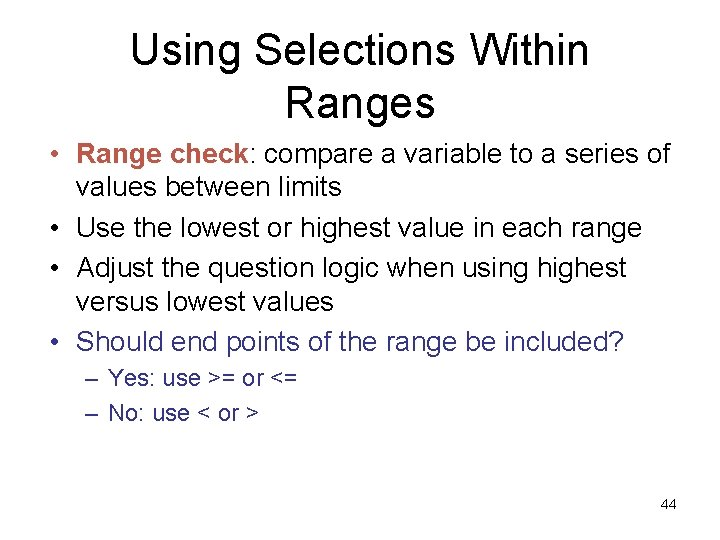Using Selections Within Ranges • Range check: compare a variable to a series of values between limits • Use the lowest or highest value in each range • Adjust the question logic when using highest versus lowest values • Should end points of the range be included? – Yes: use >= or <= – No: use < or > 44Range Selection Task: print if emp. Rate is 10 or 11 45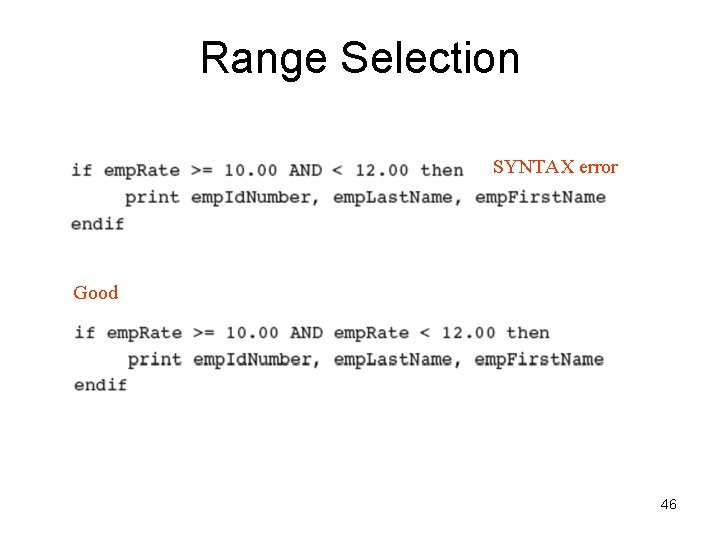Range Selection SYNTAX error Good 46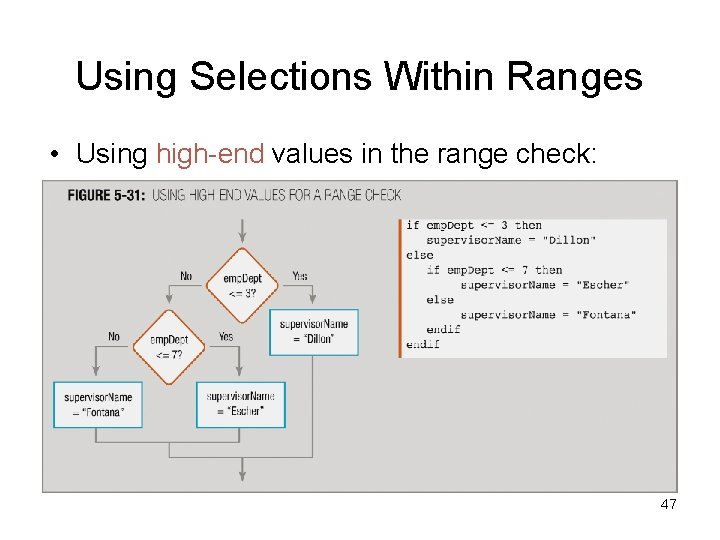Using Selections Within Ranges • Using high-end values in the range check: 47Using Selections Within Ranges • Using low-end values in the range check: 48Common Errors Using Range Checks Unnecessary 49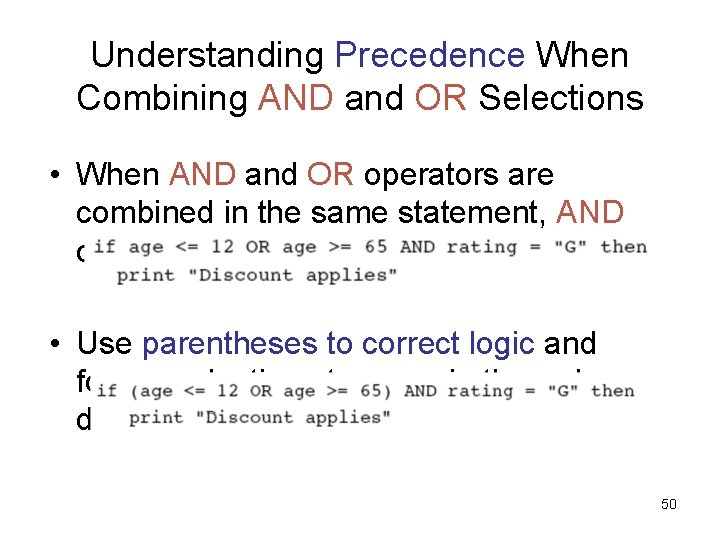Understanding Precedence When Combining AND and OR Selections • When AND and OR operators are combined in the same statement, AND operators are evaluated first • Use parentheses to correct logic and force evaluations to occur in the order desired 50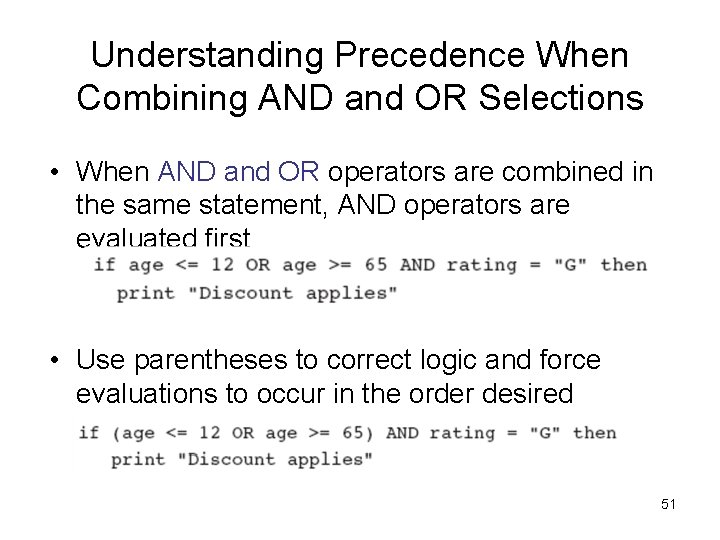Understanding Precedence When Combining AND and OR Selections • When AND and OR operators are combined in the same statement, AND operators are evaluated first • Use parentheses to correct logic and force evaluations to occur in the order desired 51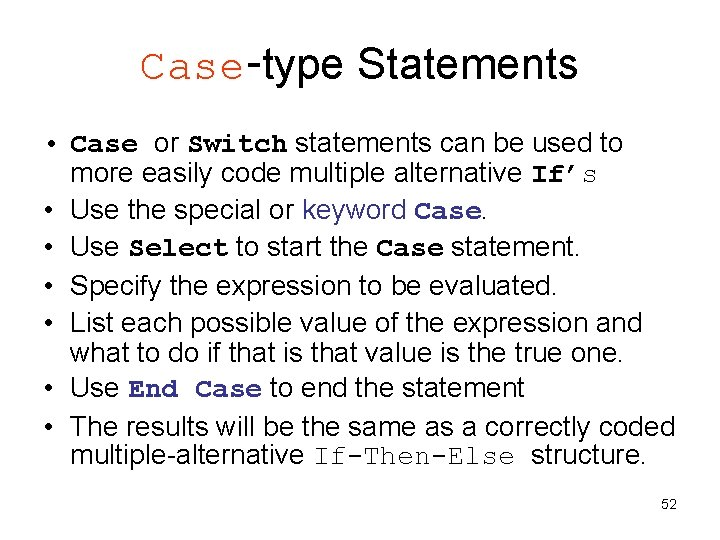Case-type Statements • Case or Switch statements can be used to more easily code multiple alternative If’s • Use the special or keyword Case. • Use Select to start the Case statement. • Specify the expression to be evaluated. • List each possible value of the expression and what to do if that is that value is the true one. • Use End Case to end the statement • The results will be the same as a correctly coded multiple-alternative If-Then-Else structure. 52Understanding the Case Structure • Used to provide a series of alternatives (IF statements) based on the value of a single variable • Replaces a series of chained if-else statements • May make the code easier to read 53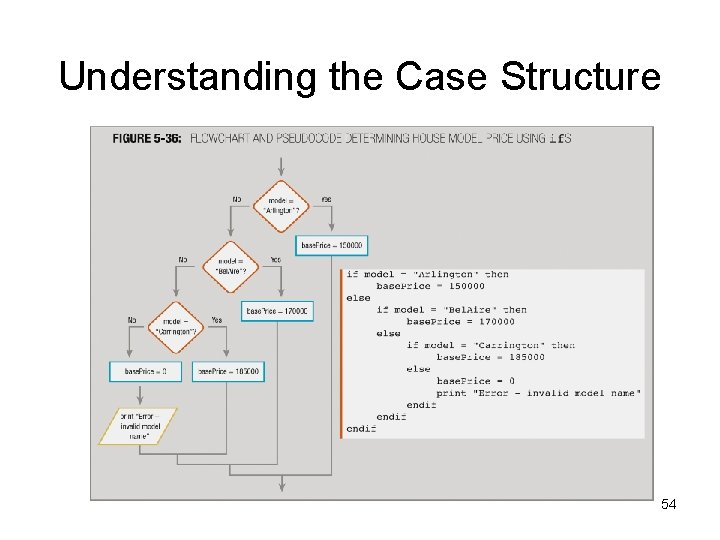Understanding the Case Structure 54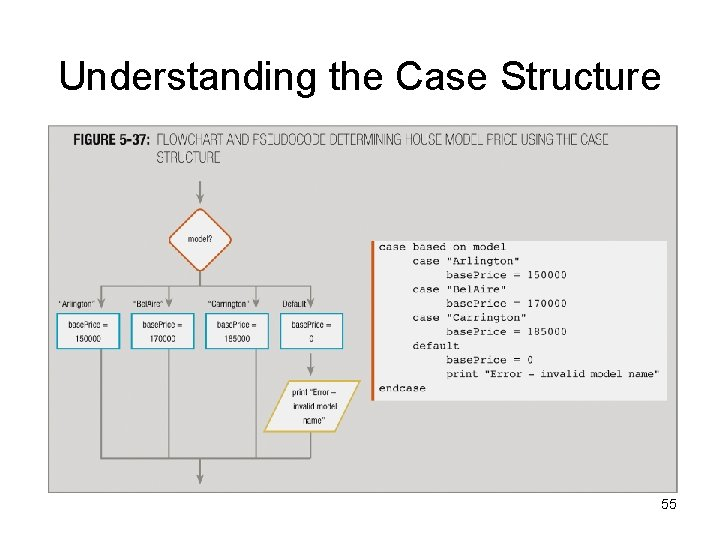Understanding the Case Structure 55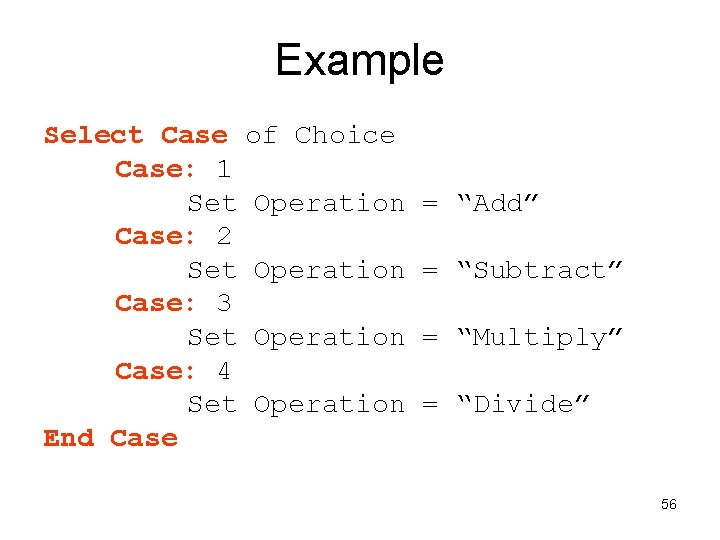Example Select Case of Choice Case: 1 Set Operation Case: 2 Set Operation Case: 3 Set Operation Case: 4 Set Operation End Case = “Add” = “Subtract” = “Multiply” = “Divide” 56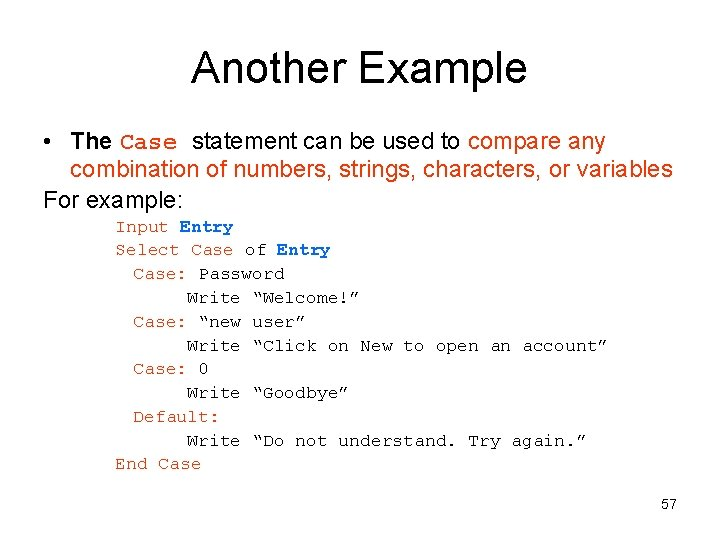Another Example • The Case statement can be used to compare any combination of numbers, strings, characters, or variables For example: Input Entry Select Case of Entry Case: Password Write “Welcome!” Case: “new user” Write “Click on New to open an account” Case: 0 Write “Goodbye” Default: Write “Do not understand. Try again. ” End Case 57• AND decision requires that both conditions be true to produce a true result • OR decision requires that either of the conditions be true to produce a true result • Case structure allows a series of alternative actions based on the value in a single variable 58Applications of Selection Structures • Program defensively in order to prevent bad data from entering our program. To do this, set error traps (idiot proofing). • If our program should accept only a Cost greater than 0, we can stop any other value from entering with the following trap: Input Cost If Cost <= 0 Then Write “Invalid cost” Else Write “The cost is ”, Cost End If 59Defensive Programming • Be sure to test your program by ‘playing computer. ’ (Deskchecking) • Perform all calculations multiple times manually • Use data that will show the results when each branch of each selection structure is executed at least once. • Check for division by zero, negative values, Nulls and other special conditions. 60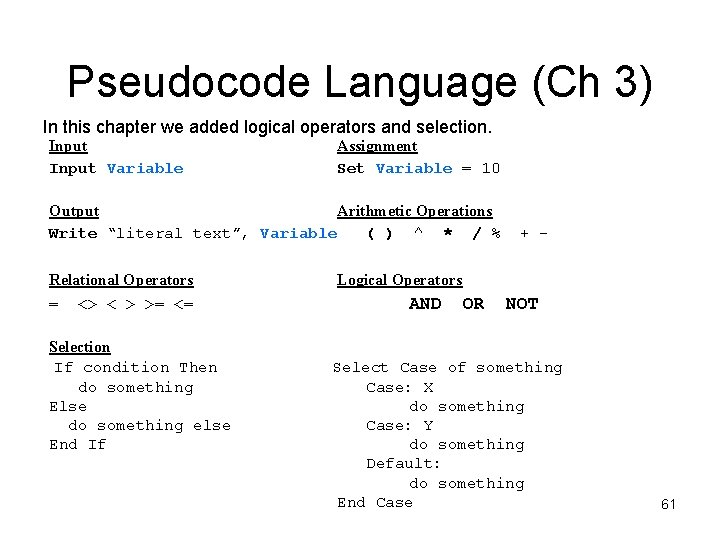Pseudocode Language (Ch 3) In this chapter we added logical operators and selection. Input Variable Assignment Set Variable = 10 Output Arithmetic Operations Write “literal text”, Variable ( ) ^ * / % Relational Operators = <> < > >= <= Selection If condition Then do something Else do something else End If + - Logical Operators AND OR NOT Select Case of something Case: X do something Case: Y do something Default: do something End Case 61